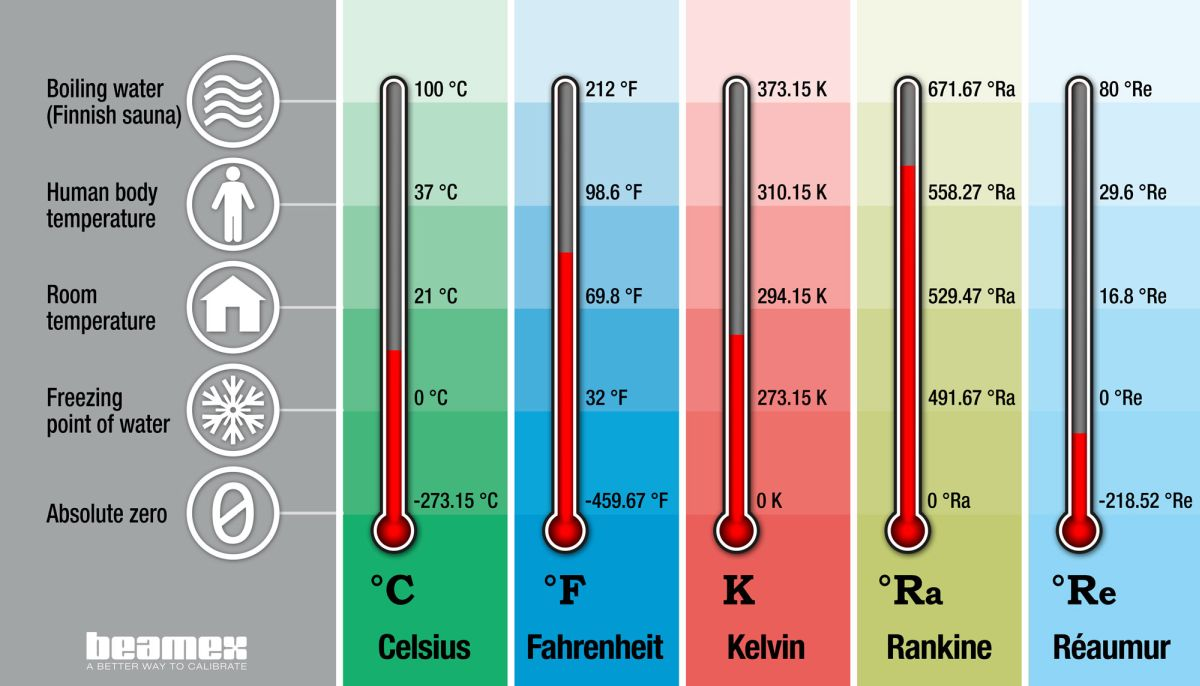Physics Laws

Beautiful Demo of PV=nRT (Boyles and charles laws and Gussacs(PV)/T = nR = constant

• when, (PV/T) is a constant,   we can compare a system before and after the changes in P, V and/or T:One mole of an ideal gas, at one degree kelvin and under one atmosphere of pressure will take up one liter.  The constant is just an empirical value that connects all those units.

Force = mass * acceleration

pressure = force / area

density = mass/ volume

volume is

Equal volumes of four different gases at the same temperature and pressure contain the same number of gaseous particles. Because the molar mass of each gas is different, the mass of each gas sample is different even though all contain 1 mol of gas.

PV=nRT

Volume increases with increasing temperature or amount (moles)  but decreases with increasing pressure.

25 x 4 = 20x1x5

50 x 2 = 20x1x5

100 x 1 = 20x1x5

volume and temperature

Nitrogen and oxygen have strong inter molecular forces

Intermolecular forces exist between nitrogen and nitrogen, oxygen and oxygen, and oxygen and nitrogen. Intermolecular forces also exist between helium and helium. Basically, any two distinct molecules will experience forces of attraction (and repulsion) between each other. The condensation point of a gas is indicative of the strength of intermolecular forces between the molecules in a gas: The lower you must bring the temperature to condense a gas, the weaker the intermolecular forces between the molecules in the gas. The condensation point of helium is 4K (-273 degrees Celsius), the condensation point of nitrogen is 77K (-196 C), the condensation point of oxygen is 90K (-183C), and the condensation point of air is 80K (-194C). In this experiment, what makes the air behave non-ideally is the fact that it is brought below its condensation point. When this is done, the air becomes a liquid! Naturally, then, it won't behave as a gas in this circumstance. Thus, any gas brought close to or below its condensation point will behave non-ideally.

Zero degrees Celsius is now defined as 273.15K. As one degree Celsius is equal to one Kelvin, boiling point of water is equal to 273.15 + 100 = 373.15 Kelvin.# Kinetic Molecular Theory and the Ideal Gas Laws

• The force of gravity, g = 9.8 m/s2
Gravity accelerates you at 9.8 meters per second per second. After one second, you're falling 9.8 m/s. After two seconds, you're falling 19.6 m/s, and so on.